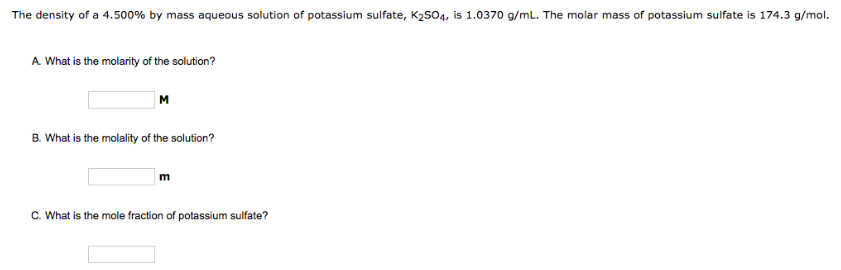# Problem: The density of a 4.500% by mass aqueous solution of potassium sulfate, K2SO4, is 1.0370 g/mL. The molar mass of potassium sulfate is 174.3 g/mol. A. What is the molarity of the solution? B. What is the molality of the solution? C. What is the mole fraction of potassium sulfate?

###### FREE Expert Solution
97% (354 ratings)###### Problem Details

The density of a 4.500% by mass aqueous solution of potassium sulfate, K2SO4, is 1.0370 g/mL. The molar mass of potassium sulfate is 174.3 g/mol.

A. What is the molarity of the solution?

B. What is the molality of the solution?

C. What is the mole fraction of potassium sulfate?